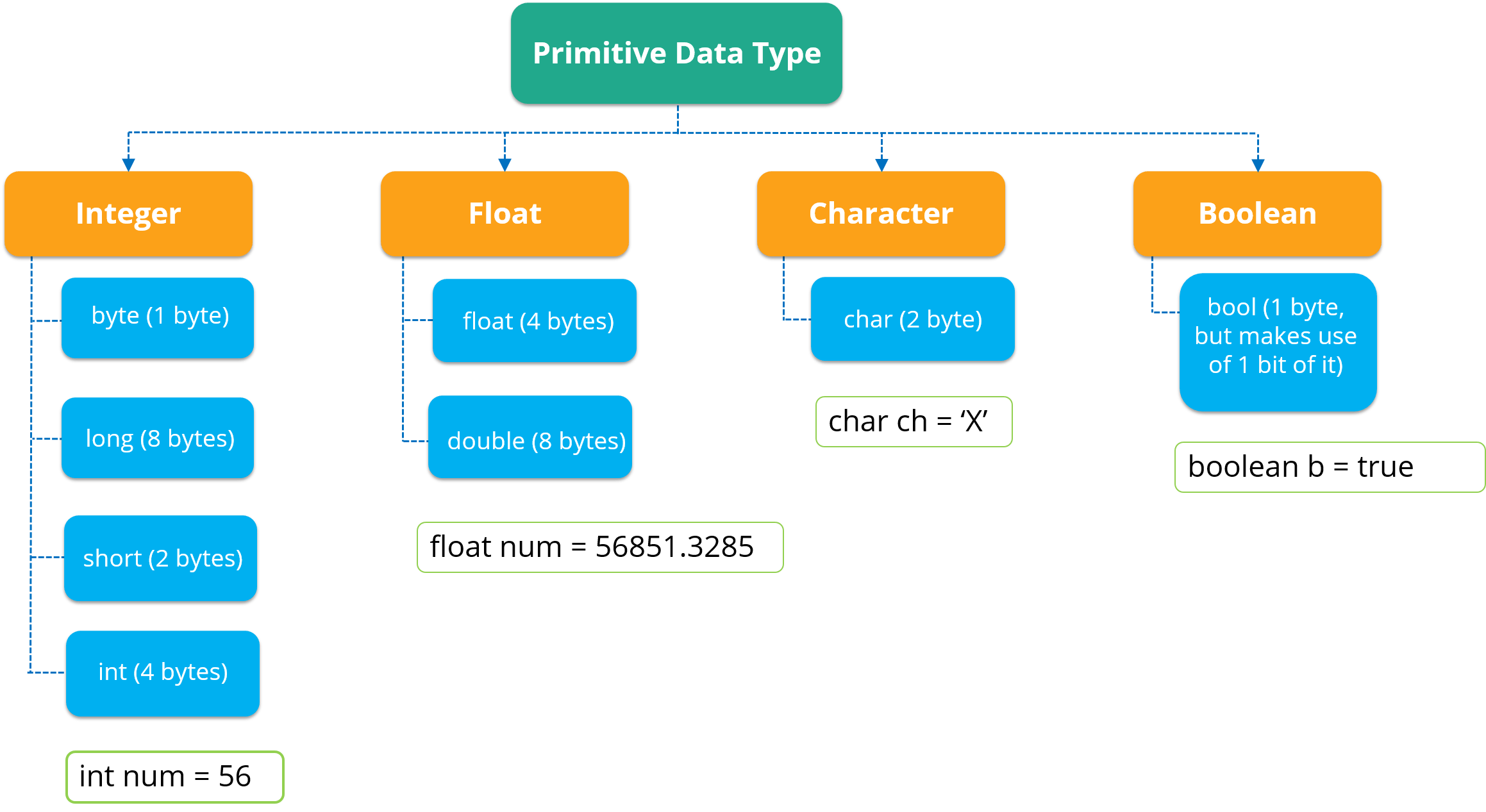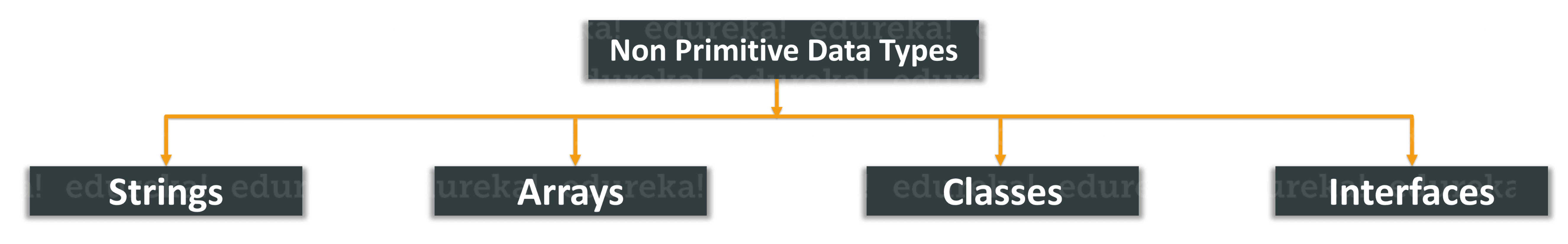# Know All About the Various Data Types in Java

Last updated on Jun 17,2021 57.2K ViewsA tech enthusiast in Java, Image Processing, Cloud Computing, Hadoop.
27 / 72 Blog from Java Core Concepts

A data type is an attribute of a variable which tells the compiler or interpreter how the programmer intends to use the variable. It defines the operations that can be done on the data and what type of values can be stored. In this article, I will give you a brief insight into the different data types in Java. According to the properties they possess, data types are divided into two groups:

Primitive Data Types: A primitive data type is pre-defined by the programming language. The size and type of variable values are specified, and it has no additional methods.

Non-Primitive Data Types: These data types are not actually defined by the programming language but are created by the programmer. They are also called “reference variables” or “object references” since they reference a memory location which stores the data.

Now, let’s move further and get into the details of Primitive Data Types.

## Primitive Data Types

Data types in Java are classified into 4 aspects as int, float, character and boolean. But, in general, there are 8 data types. They are as follows:

You can refer to the below figure to understand the different data types with respect to the memory allocated to them.Now let’s understand each of these data types in depth. First I will tell you what is boolean data type.

## boolean data type

A boolean data type comprises of a bit of information and can store only true or false values. This data type is used to track true/false conditions. Now let’s write a small program and understand how it works.

```class booleanDataType{
public static void main(String args[]){
// Setting the values for boolean data type

boolean Java = true;
boolean Python = false;
System.out.println(Java);   // Output will be true
System.out.println(Python);  // Output will be false
}
}```

That was all about the boolean data type. I hope you understood it. Now let’s move further and understand the next data type i.e. byte data type.

## byte data type

This is an example of a primitive data type. It is an 8-bit signed two’s complement integer. It stores whole numbers that lie between -128 to 127. A byte data type is helpful for saving memory in large amounts. Now let’s write a small program and understand how it works.

```class ByteExample {
public static void main(String[] args) {
byte n, a;
n = 127;
a=177;
System.out.println(n); // prints 127
System.out.println(a); // throws an error because it cannot store more than 127 bits
}
}```

That was all about the byte data type. Now let’s move further and comprehend the following data type i.e. char.

## char data type

This data type is used to store a single character. The character must be enclosed within single quotes, like ‘E’ or ‘e’. Alternatively, you can also use ASCII values to display certain characters. Let’s take a small example and see how it works.

```char  alpha = 'J';

char a = 65, b = 66, c = 67;
System.out.println(alpha); // prints J

System.out.println(a); // Displays 65
System.out.println(b); // Displays 66
System.out.println(c); // Displays 67```

That was all about the char data type. I hope you understood it. Now let’s move further and understand the next data type on the list i.e. short data type.

## short data type

A short data type is greater than byte in terms of size and less than a integer. It stores the value that ranges from  -32,768 to 32767. The default size of this data type: 2 bytes. Let’s take an example and understand the short data type.

```class ShortExample {
public static void main(String[] args) {
short n= 3435,
System.out.println(n); // prints the value present in n i.e. 3435
}
}```

Moving ahead, let’s move further and look at the next data type i.e. int data type

## int data type

This data type can store whole numbers from -2147483648 to 2147483647. Generally, int is the preferred data type when you create variables with a numeric value.

For example:

```int num = 5464564;
System.out.println(num); // prints 5464564
```

Having understood this, now let’s see which is the next data type in the list.

## long data type

This data type is a 64-bit two’s complement integer. By default, the size of a long data type is 64 bit and its value ranges from -263 to 263-1.

For example:

```long num = 15000000000L;
System.out.println(num); // prints 15000000000
```

That was all about the long data type. Now let’s move and see floating data types.

## Floating Datatypes

You should use a floating point type whenever you need a number with a decimal, such as 8.88 or 3.14515.

### float data type

This data type can store fractional numbers from 3.4e−038 to 3.4e+038. Note that you should end the value with an “f”. Let’s take a small example and understand this data type in a detailed manner.

```float num =67;
System.out.println(num); // prints the floating number value ```

So this is how you can use the float data type. Now let’s see one more floating data type i.e. double.

### double data type

The double data type can store fractional numbers from 1.7e−308 to 1.7e+308. Note that you should end the value with a “d”:

```double num = 79.678d;
System.out.println(num); // prints double value ```

That was all about Double data type and this brings us to the end of Primitive Datatypes. Now let’s figure out the difference between primitive and non-primitive data types.

## Non-Primitive Datatypes

Non-Primitive data types refer to objects and hence they are called reference types. Examples of non-primitive types include Strings, Arrays, Classes, Interface, etc. Below image depicts various non-primitive data types.Let’s now understand these non-primitive data types in short.

Strings: String is a sequence of characters. But in Java, a string is an object that represents a sequence of characters. The java.lang.String class is used to create a string object. If you wish to know more about Java Strings, you can refer to this article on Strings in Java.

Arrays: Arrays in Java are homogeneous data structures implemented in Java as objects. Arrays store one or more values of a specific data type and provide indexed access to store the same. A specific element in an array is accessed by its index. If you wish to learn Arrays in detail, then kindly check out this article on Java Arrays.

Classes: A class in Java is a blueprint which includes all your data.  A class contains fields(variables) and methods to describe the behavior of an object.

Interface: Like a class, an interface can have methods and variables, but the methods declared in interface are by default abstract (only method signature, no body).

So that was all about the non-primitive data types. Now let’s understand the difference between primitive and non-primitive data types.

### Difference between primitive and non-primitive data types

The  difference between primitive and non-primitive data types are as follows:

• Primitive types are predefined in Java. Non-primitive types are created by the programmer and is not defined by Java.
• Non Primitive types can be used to call methods to perform certain operations, while primitive types cannot.
• A primitive type always has a value, whereas non-primitive types can be null.
• A primitive type starts with a lowercase letter, while non-primitive types start with an uppercase letter.
• The size of a primitive type depends on the data type, while non-primitive types have all the same size.

This brings us to the end of the article on Data types in Java.  I hope you found it informative.

Check out the Java Certification Training by Edureka, a trusted online learning company with a network of more than 250,000 satisfied learners spread across the globe. We are here to help you with every step on your journey, for becoming a besides this java interview questions, we come up with a curriculum which is designed for students and professionals who want to be a Java Developer.

Got a question for us? Please mention it in the comments section of this “Data types in Java ”article and we will get back to you as soon as possible.REGISTER FOR FREE WEBINARThank you for registering Join Edureka Meetup community for 100+ Free Webinars each month